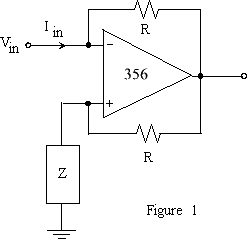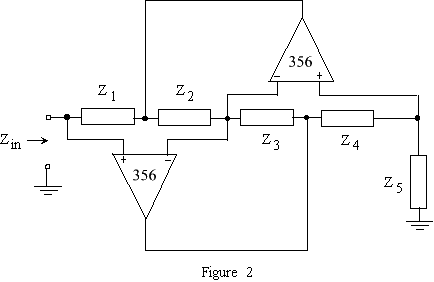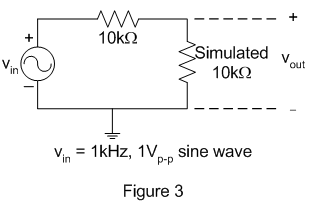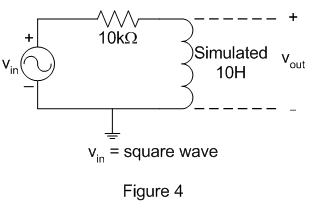EE 212 Lab
Lab 11: Operational Amplifiers,
Negative-Impedance Converters and General Impedance Converters

Note: 741 op-amps are more forgiving and work better for this experiment

This lab demonstrates two novel uses of op-amps: negative impedance converters (NICs) and general impedance converters (GICs). To understand how these circuits work, you need to analyze them in sinusoidal steady-state operation or using frequency-domain methods.

1. Negative-Impedance Converters

• The NIC shown in figure 1 uses a 356 op-amp and resistors to produce an input impedance of Zin = -Z.  Design and build a NIC with Zin = -10kW. Choose the resistors R to keep the op-amp supply current from exceeding its maximum for the rated range of input voltages. Also keep in mind that the op-amp circuit only acts as a NIC so long as it is not operating in saturation.• Put a voltage source and two 10kW resistors in series with your -10kW NIC and take measurements to verify that the NIC really behaves as -10kW. You'll want to take voltage measurements at points in the circuit other than the op-amp inputs to minimize ringing that can be caused by connection of a probe.

2. General Impedance Converters

• Figure 2 shows a GIC which is built using two op-amps and five impedances. The input impedance of a GIC is given by

Z1 Z3 Z5/(Z2 Z4).• Build a GIC to simulate (act as) a 10kW resistor using two 356 op-amps and five resistors.
• Use this simulated resistor in series with a real 10kW resistor to build a voltage divider as shown in figure 3. Drive the voltage divider with a 1kHz, 1V peak-to-peak sine wave. Does the output look like what you would expect?• A typical use of GICs is to simulate inductors. If Z2 or Z4 is a capacitor and the other impedances are resistors, then Zin is the impedance of an inductor. GICs are used to simulate inductors because it is virtually impossible to build an inductor as part of an integrated circuit, whereas op-amps, capacitors and resistors are relatively easy to build. Modify your GIC such that it acts like a 10H inductor.
• Use your "ideal'' 10H inductor in the RL circuit shown in figure 4. Determine the circuit's time constant t. (One method of finding t is to use an input square wave of period 10t to represent a step input and measure the 10% to 90% rise time from which t can be computed as in lab 3.)
• Show that this is the time constant you would expect if the inductor and resistor were both ideal.
• Increase the amplitude of the input voltage until the output becomes distorted. Why does the response of the circuit with the simulated inductor depart from the ideal response?